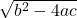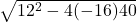After how many seconds will the ball hit the ground? h = -16t^2 + 12t + 40

Question

After how many seconds will the ball hit the ground? h = -16t^2 + 12t + 40

in progress 0
5 months 2021-08-26T19:49:36+00:00 1 Answers 5 views 0

2 seconds

Step-by-step explanation:

h = -16t^2 + 12t + 40

by the time the ball hit the ground, height (h) = 0

0 = -16t^2 + 12t + 40

solve time (t) using quadratic equation:  ax^2+bx+c=0

– b ±x = —————————–

2a

where:

a = -16

b = 12

c = 40

plugin values into the formula

– 12 ±t = ———————————-

2 (-16)

t = -5/4 , t = 2

therefore, the ball will hit the ground in 2 seconds